# Unknown number

I think number. If subtract from the twelfth square the ninth square I get a number 27 times greater than the intended number. What is this unknown number?

Result

n1 =  0
n2 =  1.405

#### Solution:Leave us a comment of example and its solution (i.e. if it is still somewhat unclear...):

Showing 0 comments:Be the first to comment!#### To solve this verbal math problem are needed these knowledge from mathematics:

Do you have a linear equation or system of equations and looking for its solution? Or do you have quadratic equation?

## Next similar examples:

1. Theorem proveWe want to prove the sentence: If the natural number n is divisible by six, then n is divisible by three. From what assumption we started?
2. Balls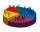We have n identical balls (numbered 1-n) is selected without replacement. Determine 1) The probability that at least one tensile strength number coincides with the number of balls? 2) Determine the mean and variance of the number of balls, which coincides.
3. Linear imaginary equationGiven that ? "this is z star" Find the value of the complex number z.
4. Ball gameRichard, Denis and Denise together scored 932 goals. Denis scored 4 goals over Denise but Denis scored 24 goals less than Richard. Determine the number of goals for each player.
5. Proof I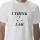When added to the product of two consecutive integers larger one, we get square larger one. Is this true or not?
6. EquationHow many real roots has equation ? ?
7. AsymptoteWhat is the vertical asymptote of ?
8. Value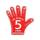Find the value of the expression: 6!·10^-3
9. GP - 8 itemsDetermine the first eight members of a geometric progression if a9=512, q=2
10. Solve 3Solve quadratic equation: (6n+1) (4n-1) = 3n2
11. Five membersWrite first 5 members geometric sequence and determine whether it is increasing or decreasing: a1 = 3 q = -2
12. Geometric sequence 4It is given geometric sequence a3 = 7 and a12 = 3. Calculate s23 (= sum of the first 23 members of the sequence).
13. Inverse matrix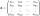Find how many times is the larger determinant is the matrix A, which equals 9 as the determinant of its inverse matrix.
14. Tenth memberCalculate the tenth member of geometric sequence when given: a1=1/2 and q=2
15. The determinantThe determinant of the unit matrix equals 7. Check how many rows the A matrix contains.
16. Intercept with axisF(x)=log(x+4)-2, what is the x intercept
17. Powers 3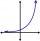2 to the power of n divided by 4 to the power of -3 equal 4. What is the vaule of n?